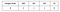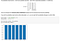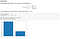# What is a Probability Distribution?

Probability distributions are an integral concept in probability theory and statistics. It is of vast importance for aspiring data scientists to solidify their understanding of probability distributions in order to be more efficacious. This article will serve as an introduction to the concept of a probability distribution.

Before delving into what a probability distribution is, there are prerequisite concepts that have to be initially understood:

• Discrete Data
• Continuous Data
• Random Variable

After defining these three terms the concepts that will be covered in the scope of this article are:

• Probability Distribution
• Discrete Probability Distribution
• Probability Mass Function
• Continuous Probability Distribution
• Probability Density Function

Discrete Data

The term “Discrete” can be defined as “separate”, “distinct”, or “detached”. Discrete data can only take on particular values. Each value is distinct and there’s no grey area in between. Discrete data can be numeric — like numbers of apples — but it can also be categorical — like red or blue, or male or female, or good or bad. There are two questions you can ask yourself when deciding if data is discrete:

• Can you count it?
• Can it be divided into smaller and smaller parts?

If you can count it then it is typically discrete and if it can be partitioned into smaller and smaller constituents then it is not discrete. Discrete data cannot be measured. For example, since you measure your weight on a scale, it’s not discrete data. Neither is the length of an object, as you use a ruler to measure it. A collection of exam grades, within a certain class, is an example of discrete data. The data can only take on a certain number of values and the number of data points are finite.

Continuous Data

If a data point can take on any value between two specified values, it is considered to be continuous. Continuous data is often measurements on a scale, such as height, weight, and temperature. Continuous data is not restricted to defined separate values, but can occupy any value over a continuous range. Between any two continuous data values, there may be an infinite number of others. Continuous data is always essentially numeric.

Random Variable

A random variable or stochastic variable can be conceptualized informally as a variable whose values depend on outcomes of a random phenomenon. It is a way to map outcomes of random processes to numbers. In other words we are quantifying outcomes by mapping them to a number. For example, we can define a random variable X in which X =1 if we toss a fair coin and it lands on heads and X = 0 if it lands on tails. In statistics, we deem phenomenon to be random if individual outcomes are uncertain but there in nonetheless a regular distribution of outcomes in a large number of repetitions. “Random” in statistics is not a synonym for “haphazard” but a description of a kind of order that emerges only in the long run. Random variables are a useful mechanism to assign probabilities to sample outcomes. Suppose that to each point of a sample space we assign a number. We then have a function defined on the sample space. This function, called a random variable, is usually denoted by a capital letter such as X or Y.

Suppose that a coin is tossed twice so that the sample space is S {HH, HT, TH, TT}. Let X represent the number of heads that can come up. With each sample point we can associate a number for X as shown in Table 2–1. Thus, for example, in the case of HH (i.e., 2 heads), X =2 while for TH (1 head), X =1. It follows that X is a random variable.Table

Suppose X denotes the number of allergic reactions among a set of eight adults. Then X is said to be a random variable and the number 3 is the value of the random variable for the outcome (yes, no, no, yes, no, no, yes, no).In general, random variables are functions that associate numbers with some attribute of a sample outcome that is deemed to be especially important. If X denotes the random variable and s denotes a sample outcome, then X (s ) = t , where t is a real number. For the allergy example, s = (yes, no, no, yes, no, no, yes, no) and t = 3.

Random variables can often create a dramatically simpler sample space. That certainly is the case here — the original sample space has 256 ( 2^8 ) outcomes, each being an ordered sequence of length eight. The random variable X, on the other hand, has only nine possible values, the integers from 0 to 8, inclusive.

In terms of their fundamental structure, all random variables fall into one of two broad categories, the distinction resting on the number of possible values the random variable can equal. If the latter is finite or countably infinite (which would be the case with the allergic reaction example), the random variable is said to be discrete; if the outcomes can be any real number in a given interval, the number of possibilities is uncountably infinite, and the random variable is said to be continuous.

• Probability Distributions

A probability distribution is a function that describes the likelihood of obtaining the possible values that a random variable can assume. In other words, the values of the variable vary based on the underlying probability distribution. When it comes to probability distributions (or probability functions) they are either discrete or continuous.

• Discrete Probability Distributions

Suppose that S is a finite or countably infinite sample space. Let p be a real-valued function defined for each element of S such that

a) 0 ≤ p(s)for each sS

b) ∑ p(s) = 1

Then p is said to be a discrete probability function

The sum of all probabilities for all possible values must equal 1 and no value can be negative. Furthermore, the probability for a particular value or range of values must be between 0 and 1. A discrete probability function is also called a probability mass function ( or PMF).Discrete Uniform Distribution in Python

The python code above showcases a discrete probability distribution known as a uniform distribution. A uniform distribution is one in which each sample outcome has the equal probability. When it comes to rolling dice, there are six equally likely outcomes. Therefore the probability distribution only takes on the probability 1/6 for each sample outcome.

Another example of a discrete probability distribution is a Bernoulli Distribution also called: Bernoulli Trial.Bernoulli Trial in Python

Continuous Distribution

A probability function P on a set of real numbers S is called continuous if there exists a function f(x) such that for any closed interval [a,b] ⊂ S , P[(a,b)] = the definite integral from a to b.

A probability distribution in which the random variable X can take on any value is continuous. Because there are infinite values that X could assume, the probability of X taking on any one specific value is zero. Therefore we often speak in ranges of values (p(X>0) = .50). An equation or formula is used to describe a continuous probability distribution.The equation used to describe a continuous probability distribution is called a probability density function (pdf). All probability density functions satisfy the following conditions:

• The random variable Y is a function of X; that is, y = f(x).
• The value of y is greater than or equal to zero for all values of x.
• The total area under the curve of the function is equal to one.

Conclusion

The probability distributions are a common way to describe, and possibly predict, the probability of an event. The main point is to define the character of the variables whose behavior we are trying to describe. The identification of the right category will allow a proper application of a model (for instance, the standardized normal distribution) that would easily predict the probability of a given event. Grasping the concept of probability distributions is a crucial predecessor to possessing the capability of statistical analysis and modeling.

## More from Shiffraw dagnechaw

Data Science Enthusiast